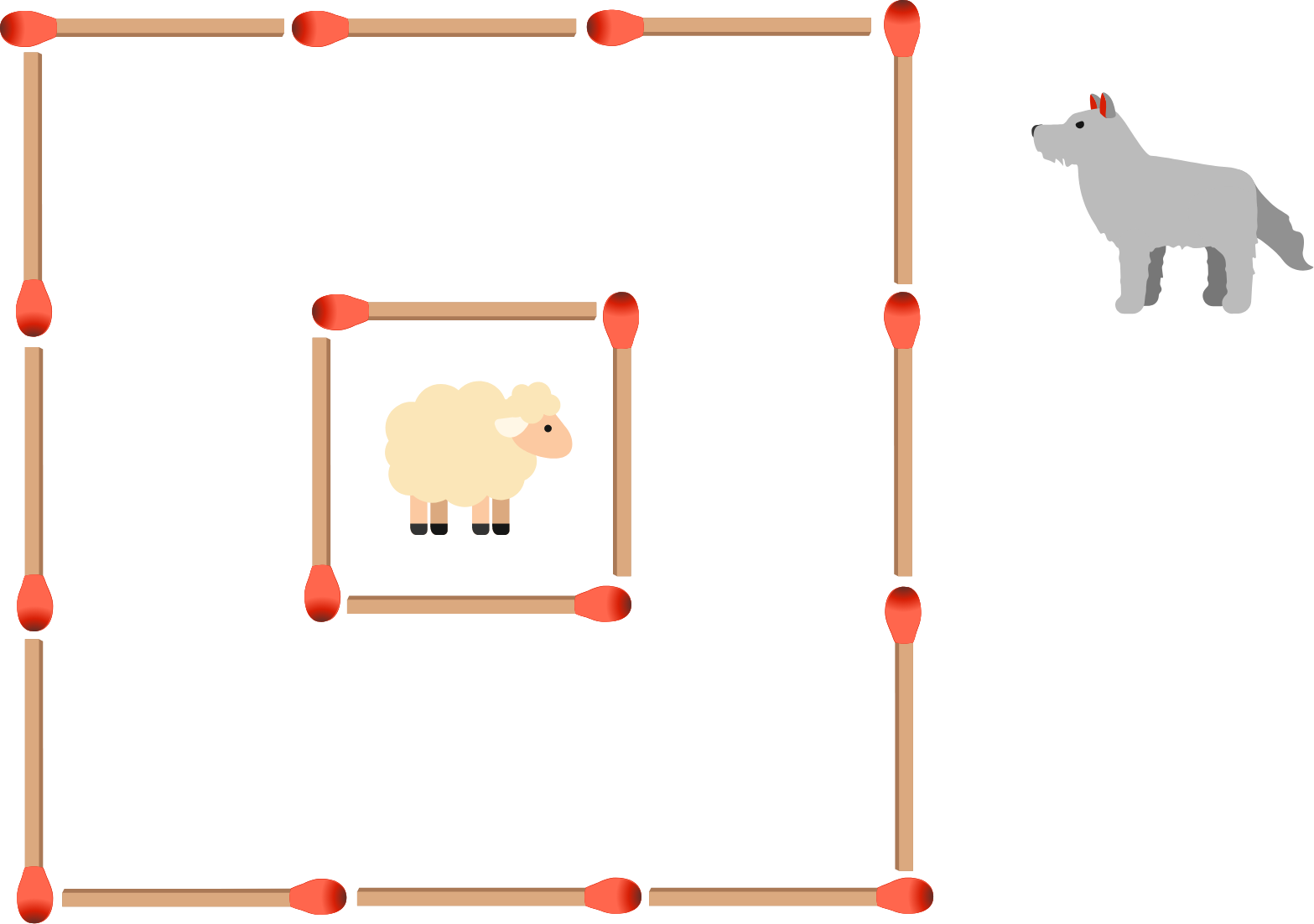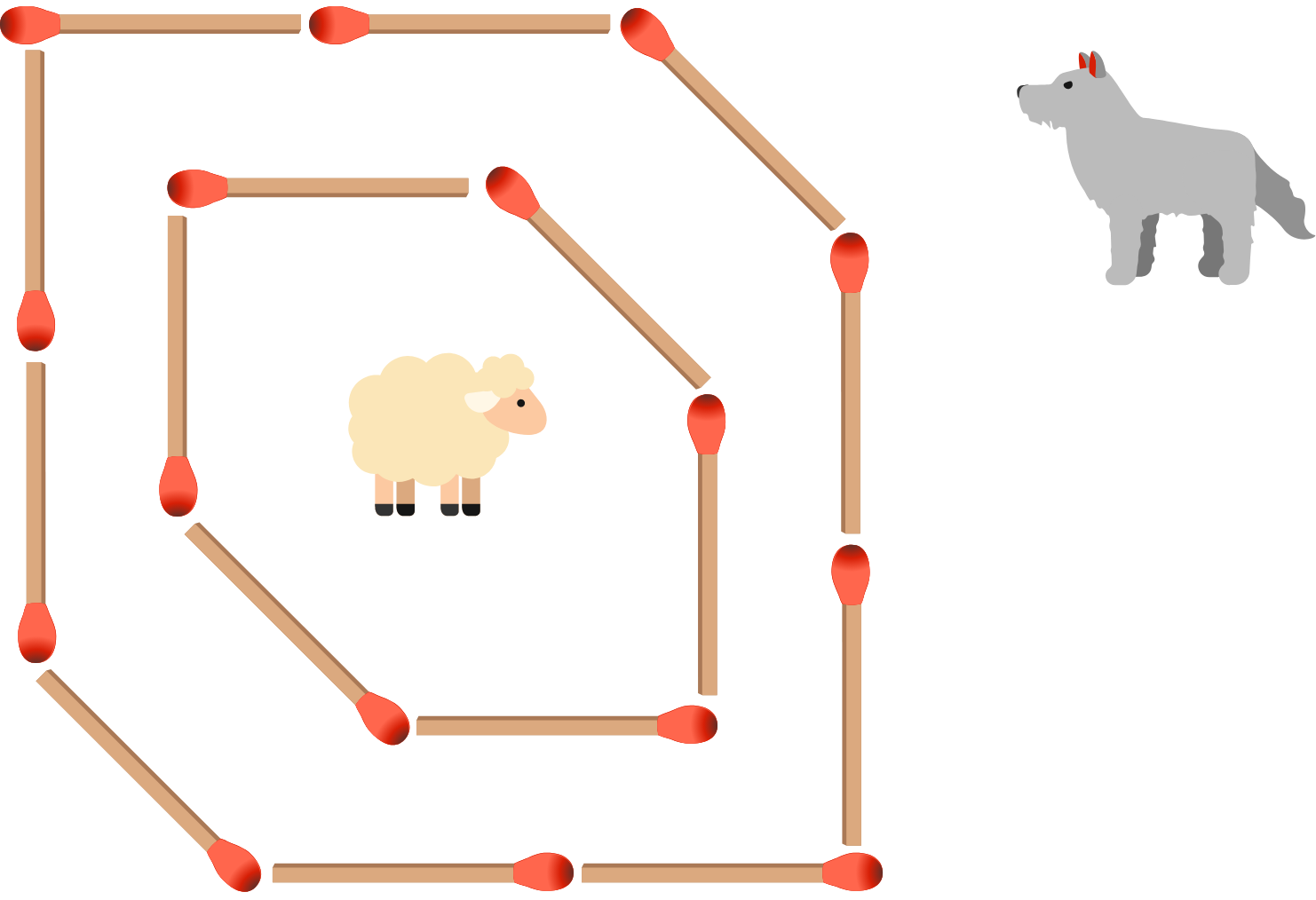Perplexing ProbabilityAll of the techniques and mathematical tools we'll introduce can also be applied to many situations outside of puzzles. Probability problems appear everywhere and every day, yet the next $\textbf5$ problems in this quiz are practical cases that trick even experienced problem-solvers!

Probability is EverywhereOne day, the traffic light at a certain intersection is red when you reach it and no other cars are in front of you. You wait $45$ seconds for it to turn green.

The next day you reach the same light and it's red again. Additionally, another car is already there, waiting for the light to change, and you pull to a stop behind him.

Which scenario is more likely to occur on the second day?

NOTE: You may assume the light changes at regular intervals and is not affected by when cars are near or based on the time of day.

Probability is EverywhereSuppose you have a bag with $5$ chocolate caramels and $5$ cherry cordials. Your friend grabs a candy at random and eats it. Afterwards, without looking at what's left, you grab and eat one of the remaining $9$ candies. What is the probability you just ate a cherry cordial?

Probability is Everywhere

Probability in Social ScienceThe world's oldest Olympic medalist was $72$ years old (Oscar Swahn of Sweden). Pictured above is Amy. Which of these statements about her is more likely to be true?

Probability is Everywhere

Probability in Science and Health

For this puzzle, test your rapid-fire intuition by guessing without calculating, or do the calculation if you want.

Disease Z infects $1$ out of every $1000$ people. There's a test for Disease Z which is guaranteed to test positive for someone with the disease. For those without the disease, they will test positive $1\%$ of the time.

You tested positive for Disease Z, and your doctor wants to place you on an expensive emergency treatment. What's the probability you actually have it?Probability is Everywhere

Probability in Engineering and Product DesignThe matchsticks represent fences protecting a sheep from a wolf. Note that if two of the matchsticks are removed at random, then the wolf could get to the sheep if one removal is from the outside fence and one from the inside fence.

Suppose we move two matchsticks from the outer ring to the inner ring, as shown below. Given two matchsticks will then still be removed at random, is the new configuration any safer than the original?×